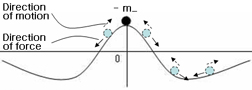Why dark matter does not emit photon?
We are now living in the era of new revolution.

Are we again treading on the process of huge revolution that occurred in the late 19th century and the early 20th century?

The current observation of dark matter requires explanation on two important situations as the following.

Situation 1: Denial of the gravitational (attractive) phenomenon
Dark matter is five times more than the general matter, but it does not show macro-structure like the structure of galaxy or galaxy cluster, or micro-structure like fixed star or planet. The result of the current observation is that dark matter has attractive interaction with the general matter, but does not interact with the other dark matter. In other words, the observation or interpretation is that dark matters do not exert gravity(attraction) on each other.

Situation 2: Denial of the fundamental phenomenon of physics that absorbs or emits photons
It is not the level that dark matter has not interacts electromagnetically because it is electrically neutral, but the observation result is the level that dark matter does not emits or absorbs photons.

From the several explanations above, Situation 1 shows that the essence of dark matter is negative mass, and that consequently they have the repulsive gravitational effect, then it can be explained.

In regard to Situation 2, without giving that kind of characteristics of "the observation result that seems to be that it does not emit or absorb photons" from the first, can this kind of result be induced from other basic principle?

A. Negative mass is stable at the state of high energy.Fig1. , The acceleration of negative mass is opposite of the direction of force, and in case of negative mass, it does harmonic oscillation at the maximum point and is stable at the maximum point.

B. The problem of transition from positive energy level to negative energy level

Since we do not know clearly about the characteristics of negative mass yet, we do not know whether transition of positive mass at the positive energy level to negative energy level is possible or not.Fig2. Transition to the negative energy level( )

However, for positive mass to enter the area of negative energy level, energy should have the negative value, and this means that it should have the characteristics of the negative mass. When considering the process of entering the domain of negative energy level from positive energy level, it must pass through the domain between 0 and (-hbar/2)*w. In case it follows the laws of negative mass because it's in the domain of negative energy, it cannot reach (-hbar/2)*w ,which is the first energy level of negative, because it is stable at the state of high energy, and it tries to have higher value of energy. It is because the energy level 0 is much higher than the energy level (-hbar/2)*w . That is to say that it shows possibility that the law of negative mass itself does not allow the situation where positive mass at the positive energy level succeed to the negative energy level.

Even if it reaches (-hbar/2)*w, the first energy level, at the negative energy level, it must follow the law of negative mass, it is stable at the state of high energy, and thus "the problem of the transition of the energy level of minus infinity" does not occur.

As we have examined above, "the problem of the transition of the energy level of minus infinity" does not occur, and thus positive mass and negative mass can exist in the same spacetime. This is a very important result because it means that negative mass and negative energy can exist stably in our universe.

(This finding suggests that "the problem of the transition of the energy level of minus infinity", which we have had for eighty years regardless of whether negative mass exists or not, does not occur, and it itself has important meaning. Thus, it needs to be reflected in the teachings that are being performed when explaining the solution to negative energy, which the relativistic energy eq. suggests.)

In regard to Situation 2, without giving that kind of characteristics of "the observation result that seems to be that it does not emit or absorb photons" from the first, can this kind of result be induced from other basic principle?

C. Why dark matter does not emit photon?
1) The process of negative mass emitting photon
-E_ - hv < -E_
-E_ : Initial energy of negative mass,(E_ > 0)
hv : Energy of photon
The relational expression above means that if negative mass emits photon(with positive energy), then the energy after emitting gets lower than the energy before emitting.

Negative mass is stable at the state of high energy, so the voluntary transition that negative mass emits photon and succeeds to the lower energy level does not exist. Therefore, negative mass explains the result of the current observation that dark matter does not emit photon from the fundamental principle.

* The explanation on why dark matter does not emit photon is enough with this. In my paper, more various situations are set-up.

Negative Mass is Stable at the State of High Energy
http://vixra.org/abs/1107.0052